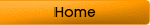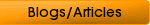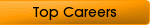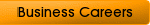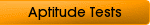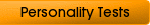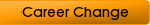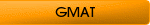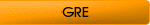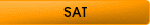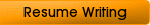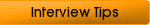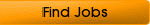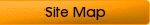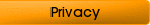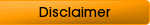## Essentials of The Numerical Test

The psychologists design numerical test from various mathematical fields including numeric operations, roman system, decimals, ratios, fractions, geometry, trigonometry, algebra etc. Every question is intended to measure your math reasoning capabilities. You are required to identify logical relationships and underlying principles in the given mathematical statements and then apply those effectively to solve the problems. You are generally offered series of numbers, multiplications and divisions to identify patterns.

But numerical tests are not meant to measure your computational skills...

...they focus upon your analytical abilities!

It means you can score well even when you are not fully skilled but possess sharp analytical mind. The numeric aptitude tests are designed in the following major areas.

### Operations of Numbers

You are offered various number problems which use different symbols. You need to understand these general symbols before attempting any numerical test. They are just a few…

≠ is not equal to

> is greater than (5 > 4)

< is lesser than (4 < 5)

≥ is greater than or equal to

≤ is lesser than or equal to

: and :: to the ratio to

√ square root of (√16 = 4)

|x| absolute value

### Operations with Complex Numbers

Complex numbers are really complex. They contain two parts; real and imaginary. While attempting a numeric aptitude test problem, you should handle the both parts effectively.

To add two complex numbers in numerical tests, you have to add their real parts and imaginary parts separately. Thus,

(a+b) + (c+d)= (a+c) +i(b+d)

For example:

(2+i) + (5+3i) = 7+4i

This way you have added two real number (2+5) and imaginary numbers (i+3i) separately.

Subtraction of Complex Numbers

To subtract one complex number from another complex number, you have to subtract real and imaginary parts of the second complex number from the real and imaginary parts of the first complex numbers, respectively. This way,

(a+ib)-(c+id) = (a-c) + i(b-d)

For example:

(2+3i) (-4+7i) = -8-21-12i+4i =-29+2i

Division of Complex Number

In numerical tests, you may be offered problems which involve division of complex numbers. However, before knowing the process, you need to know the concept of conjugate of a complex number.

If Z = x+ iy then

The conjugate of Z is equal to x-iy.

When you want to calculate a+ib/c+id, you will have to multiply the numerator and denominator by the conjugate of the denominator. Thus…

a+ib/c+id = (a+ib)(c-id)/(c+id)(c-id)

For example...

2+i/1+3i = (2-i)(1-3i)/(1+3i)(1-3i) = 2-3-i-6i/1+9 = -1/10-7i/10

Multiplication of Complex Number

When you multiply the complex numbers, the numbers multiplied are called the factors of the product and the result of the operation is called the product. For example, the numbers 4 and 4 are the factors of 16.

### Roman System

Under the Roman System of numbers you represent real numbers with roman symbols. For example, you write I for 1, V for 5, X for 10, L for 50, C for 100, D for 500 and M for 1000. A smaller number placed in left of these numbers indicates a subtraction and on the right side denotes an addition.

While performing division operations, the number which you divide is called the dividend. The number which divides is called the divisor. The number which tells how many times the divisor is contained in a dividend is called the remainder. When there is no remainder, the division is called exact division.

### Basic Algebra

Any expression which connects variables and constants by algebraic operations of addition, subtraction, multiplication, division, and root extraction is called an algebraic expression. For example:

√x + 1/√x or

4x + 2x +8

...are algebraic expressions.

You are required to use algebraic methods to investigate, model and interpret patterns and functions which involve numbers, shapes, data and graphics in the given problems. You are also required to know basic order of operations to carry out multiplications, divisions, additions and subtractions. You should learn what are Greatest Common Divisor (G.C.D), Least Common Multiple (L.C.M), exponents etc.

### Geometry

It is an interesting subject of numerical tests. You are required to know geometric concepts, properties, and relationships in a problem-solving situation. Characteristics of parallel, perpendicular and interesting lines are commonly used. The knowledge about characteristics and terminology of angles shall help you to avoid ditches. The basics of circles, perimeters, rectangular coordinator system, ordinate and point are essential to be learnt. You should study the concepts of polygon and classification (triangle, quadrilaterals, pentagons and hexagons) before encountering numerical tests in any psychological screening process.

### Measurements

You can find questions about measurements in almost all numerical tests. You are required to use a variety of tools and techniques of measurement. The problems may include time, space and weight as measurement units. Techniques of dimensional analysis may also help you to find out correct solutions of tricky questions.

### Statistics and Probability

Most of the numerical tests focus upon the questions which involve a lot of statistical data and probabilities. You are required to analyze any given situation and arrive to the conclusion with the help of your math reasoning. You should have some concept of the procedures which involve statistical data collection, organization and estimations to draw your inferences.